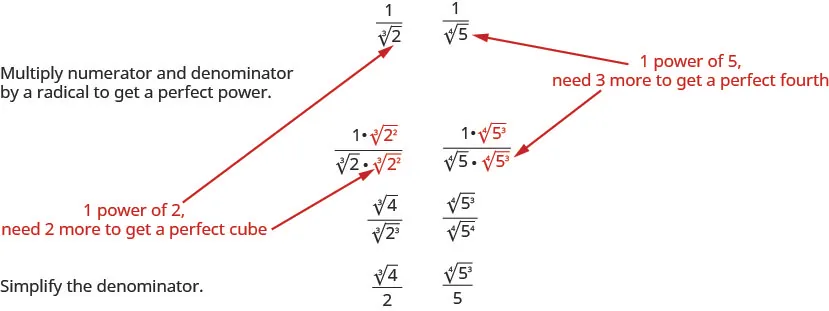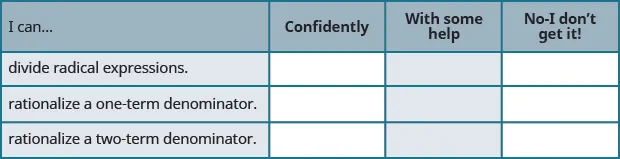Intermediate Algebra 2e

Intermediate Algebra 2e8.5 Divide Radical Expressions

### Learning Objectives

By the end of this section, you will be able to:

• Rationalize a one term denominator
• Rationalize a two term denominator

### Be Prepared 8.13

Before you get started, take this readiness quiz.

Simplify: $3048.3048.$
If you missed this problem, review Example 1.24.

### Be Prepared 8.14

Simplify: $x2·x4.x2·x4.$
If you missed this problem, review Example 5.12.

### Be Prepared 8.15

Multiply: $(7+3x)(7−3x).(7+3x)(7−3x).$
If you missed this problem, review Example 5.32.

We have used the Quotient Property of Radical Expressions to simplify roots of fractions. We will need to use this property ‘in reverse’ to simplify a fraction with radicals.

We give the Quotient Property of Radical Expressions again for easy reference. Remember, we assume all variables are greater than or equal to zero so that no absolute value bars are needed.

### Quotient Property of Radical Expressions

If $anan$ and $bnbn$ are real numbers, $b≠0,b≠0,$ and for any integer $n≥2n≥2$ then,

$abn=anbnandanbn=abnabn=anbnandanbn=abn$

We will use the Quotient Property of Radical Expressions when the fraction we start with is the quotient of two radicals, and neither radicand is a perfect power of the index. When we write the fraction in a single radical, we may find common factors in the numerator and denominator.

### Example 8.47

Simplify: $72x3162x72x3162x$ $32x234x53.32x234x53.$

### Try It 8.93

Simplify: $50s3128s50s3128s$ $56a37a43.56a37a43.$

### Try It 8.94

Simplify: $75q5108q75q5108q$ $72b239b53.72b239b53.$

### Example 8.48

Simplify: $147ab83a3b4147ab83a3b4$ $−250mn−232m−2n43.−250mn−232m−2n43.$

### Try It 8.95

Simplify: $162x10y22x6y6162x10y22x6y6$ $−128x2y−132x−1y23.−128x2y−132x−1y23.$

### Try It 8.96

Simplify: $300m3n73m5n300m3n73m5n$ $−81pq−133p−2q53.−81pq−133p−2q53.$

### Example 8.49

Simplify: $54x5y33x2y.54x5y33x2y.$

### Try It 8.97

Simplify: $64x4y52xy3.64x4y52xy3.$

### Try It 8.98

Simplify: $96a5b42a3b.96a5b42a3b.$

### Rationalize a One Term Denominator

Before the calculator became a tool of everyday life, approximating the value of a fraction with a radical in the denominator was a very cumbersome process!

For this reason, a process called rationalizing the denominator was developed. A fraction with a radical in the denominator is converted to an equivalent fraction whose denominator is an integer. Square roots of numbers that are not perfect squares are irrational numbers. When we rationalize the denominator, we write an equivalent fraction with a rational number in the denominator.

This process is still used today, and is useful in other areas of mathematics, too.

### Rationalizing the Denominator

Rationalizing the denominator is the process of converting a fraction with a radical in the denominator to an equivalent fraction whose denominator is an integer.

Even though we have calculators available nearly everywhere, a fraction with a radical in the denominator still must be rationalized. It is not considered simplified if the denominator contains a radical.

Similarly, a radical expression is not considered simplified if the radicand contains a fraction.

A radical expression is considered simplified if there are

• no factors in the radicand have perfect powers of the index
• no fractions in the radicand
• no radicals in the denominator of a fraction

To rationalize a denominator with a square root, we use the property that $(a)2=a.(a)2=a.$ If we square an irrational square root, we get a rational number.

We will use this property to rationalize the denominator in the next example.

### Example 8.50

Simplify: $4343$ $320320$ $36x.36x.$

### Try It 8.99

Simplify: $5353$ $332332$ $22x.22x.$

### Try It 8.100

Simplify: $6565$ $718718$ $55x.55x.$

When we rationalized a square root, we multiplied the numerator and denominator by a square root that would give us a perfect square under the radical in the denominator. When we took the square root, the denominator no longer had a radical.

We will follow a similar process to rationalize higher roots. To rationalize a denominator with a higher index radical, we multiply the numerator and denominator by a radical that would give us a radicand that is a perfect power of the index. When we simplify the new radical, the denominator will no longer have a radical.

For example,We will use this technique in the next examples.

### Example 8.51

Simplify $163163$ $72437243$ $34x3.34x3.$

### Try It 8.101

Simplify: $173173$ $51235123$ $59y3.59y3.$

### Try It 8.102

Simplify: $123123$ $32033203$ $225n3.225n3.$

### Example 8.52

Simplify: $124124$ $56445644$ $28x4.28x4.$

### Try It 8.103

Simplify: $134134$ $36443644$ $3125x4.3125x4.$

### Try It 8.104

Simplify: $154154$ $7128471284$ $44x444x4$

### Rationalize a Two Term Denominator

When the denominator of a fraction is a sum or difference with square roots, we use the Product of Conjugates Pattern to rationalize the denominator.

$(a−b)(a+b)(2−5)(2+5)a2−b222−(5)24−5−1(a−b)(a+b)(2−5)(2+5)a2−b222−(5)24−5−1$

When we multiply a binomial that includes a square root by its conjugate, the product has no square roots.

### Example 8.53

Simplify: $52−3.52−3.$

### Try It 8.105

Simplify: $31−5.31−5.$

### Try It 8.106

Simplify: $24−6.24−6.$

Notice we did not distribute the 5 in the answer of the last example. By leaving the result factored we can see if there are any factors that may be common to both the numerator and denominator.

### Example 8.54

Simplify: $3u−6.3u−6.$

### Try It 8.107

Simplify: $5x+2.5x+2.$

### Try It 8.108

Simplify: $10y−3.10y−3.$

Be careful of the signs when multiplying. The numerator and denominator look very similar when you multiply by the conjugate.

### Example 8.55

Simplify: $x+7x−7.x+7x−7.$

### Try It 8.109

Simplify: $p+2p−2.p+2p−2.$

### Try It 8.110

Simplify: $q−10q+10q−10q+10$

### Media

Access these online resources for additional instruction and practice with dividing radical expressions.

### Section 8.5 Exercises

#### Practice Makes Perfect

Divide Square Roots

In the following exercises, simplify.

245.

$1287212872$ $12835431283543$

246.

$48754875$ $813243813243$

247.

$200m598m200m598m$ $54y232y5354y232y53$

248.

$108n7243n3108n7243n3$ $54y316y4354y316y43$

249.

$75r3108r775r3108r7$ $24x7381x4324x7381x43$

250.

$196q484q5196q484q5$ $16m4354m316m4354m3$

251.

$108p5q23p3q6108p5q23p3q6$ $−16a4b−232a−2b3−16a4b−232a−2b3$

252.

$98rs102r3s498rs102r3s4$ $−375y4z−233y−2z43−375y4z−233y−2z43$

253.

$320mn−545m−7n3320mn−545m−7n3$ $16x4y−23−54x−2y4316x4y−23−54x−2y43$

254.

$810c−3d71000cd−1810c−3d71000cd−1$ $24a7b−13−81a−2b2324a7b−13−81a−2b23$

255.

$56 x 5 y 4 2 x y 3 56 x 5 y 4 2 x y 3$

256.

$72 a 3 b 6 3 a b 3 72 a 3 b 6 3 a b 3$

257.

$48 a 3 b 6 3 3 a − 1 b 3 3 48 a 3 b 6 3 3 a − 1 b 3 3$

258.

$162 x − 3 y 6 3 2 x 3 y − 2 3 162 x − 3 y 6 3 2 x 3 y − 2 3$

Rationalize a One Term Denominator

In the following exercises, rationalize the denominator.

259.

$106106$ $427427$ $105x105x$

260.

$8383$ $740740$ $82y82y$

261.

$6767$ $845845$ $123p123p$

262.

$4545$ $27802780$ $186q186q$

263.

$153153$ $52435243$ $436a3436a3$

264.

$133133$ $53235323$ $749b3749b3$

265.

$11131113$ $75437543$ $33x2333x23$

266.

$11331133$ $3128331283$ $36y2336y23$

267.

$174174$ $53245324$ $44x2444x24$

268.

$144144$ $93249324$ $69x3469x34$

269.

$194194$ $251284251284$ $627a4627a4$

270.

$184184$ $271284271284$ $1664b241664b24$

Rationalize a Two Term Denominator

In the following exercises, simplify.

271.

$8 1 − 5 8 1 − 5$

272.

$7 2 − 6 7 2 − 6$

273.

$6 3 − 7 6 3 − 7$

274.

$5 4 − 11 5 4 − 11$

275.

$3 m − 5 3 m − 5$

276.

$5 n − 7 5 n − 7$

277.

$2 x − 6 2 x − 6$

278.

$7 y + 3 7 y + 3$

279.

$r + 5 r − 5 r + 5 r − 5$

280.

$s − 6 s + 6 s − 6 s + 6$

281.

$x + 8 x − 8 x + 8 x − 8$

282.

$m − 3 m + 3 m − 3 m + 3$

#### Writing Exercises

283.

Simplify $273273$ and explain all your steps.
Simplify $275275$ and explain all your steps.
Why are the two methods of simplifying square roots different?

284.

Explain what is meant by the word rationalize in the phrase, “rationalize a denominator.”

285.

Explain why multiplying $2x−32x−3$ by its conjugate results in an expression with no radicals.

286.

Explain why multiplying $7x37x3$ by $x3x3x3x3$ does not rationalize the denominator.

#### Self Check

After completing the exercises, use this checklist to evaluate your mastery of the objectives of this section.After looking at the checklist, do you think you are well-prepared for the next section? Why or why not?

Order a print copy

As an Amazon Associate we earn from qualifying purchases.